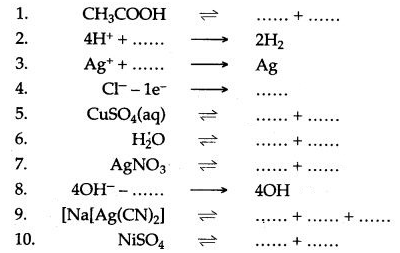ICSESolutions.com provides ICSE Solutions for Class 10 Chemistry Chapter 6 Electrolysis for ICSE Board Examinations. We provide step by step Solutions for ICSE Chemistry Class 10 Solutions Pdf. You can download the Class 10 Chemistry ICSE Textbook Solutions with Free PDF download option.

## ICSE Class 10 Chemistry Chapter 6 Electrolysis Solutions

### Short Questions

Question 1: Classify following substances under three headings:
Strong electrolytes, Weak electrolytes, Non-electrolytes.
Acetic acid, ammonium chloride, ammonium hydroxide, carbon tetrachloride, dilute hydrochloric acid, sodium acetate, dilute sulphuric acid.
Answer: Strong electrolytes —Ammonium chloride, dilute hydrochloric acid, dilute sulphuric acid.
Weak electrolytes — Ammonium hydroxide, acetic acid, sodium acetates.
Non-electrolyte — Carbon tetra chloride.

Question 2: How will you distinguish between metallic conductions and electrical conductions ?

 Metallic Conduction Electrical Conduction (i) By the Movement of electrons. By the Movement of ions. (ii) Does not involve the transfer of matter. It involves the transfer of matter as ions. (iii) No change in chemical properties of the conductor. It involve the decomposition of electrolyte as a result of chemical reactions. (iv) Increase in resistance with, die rise of temperature. Decrease in resistance with the rise of temperature.

Question 3: Differentiate between an Electrolytic cell and Electrochemical cell.

 Electrolytic cell Electrochemical cell It is a device (vessel) in which chemical changes are brought about with the help of electric energy. It is a device in which electric energy is generated as a result of chemical change.

Question 4: Differentiate between electrical conductivity of copper sulphate solution and copper metal.
Answer: Differences between electrical conductivity of copper sulphate solution and copper metal:

 Copper sulphate solution Copper metal Electric current is by flow of ions. Electric current is by flow of electrons. It is aqueous solution of ionic compound. It is a metal in solid state. Copper sulphate undergoes a chemical change. Copper metal remains unchanged chemically.

Question 5: Choose A, B, C or D to match the descriptions (i) to (v) below. Some alphabets may be repeated.
A. non-electrolyte
B. strong electrolyte
C. Weak electrolyte
D. metallic conductor
(i) Molten ionic compound
(ii) Carbon tetrachloride
(iii) An aluminium wire
(iv) A solution containing solvent molcules, solute molecules and ions formed by the dissociation of solute molecules.
(v) A sugar solution with sugar molecules and water molecules.
Answer: (i) B, (ii) A, (iii) D, (iv) C, (v) A.

Question 6: Give three differences between sodium atom and sodium ion.
Answer: (i) Sodium atom is neutral in nature, while sodium ion is a positively charged particle.
(ii) Sodium atom vigorously reacts with water to liberate hydrogen gas, while sodium ion does not react with water.
(iii) Sodium atom tends to lose an electron to form sodium ion with a complete octet in the outermost shell.

Question 7: Explain how electrolysis is an example of a redox reaction.
Answer: Redox reactions are called simultaneous oxidation-reduction reactions. In electrode reactions, the positively charged ions (cations) accept electrons from the cathode to form neutral atoms, i.e., at cathode reduction takes place. At the anode, the negatively charged ions (anions) lose electrons to form neutral atoms, i.e., anode oxidation takes place. So, electrode reactions also signify oxidation-reduction reactions. Hence, they are also called Redox reactions.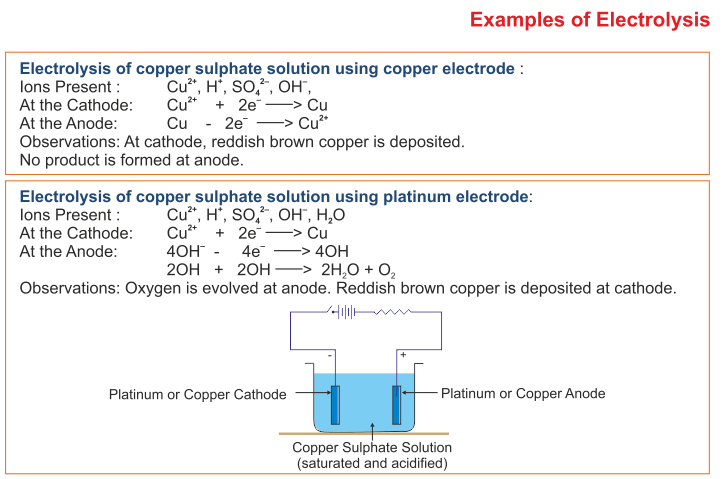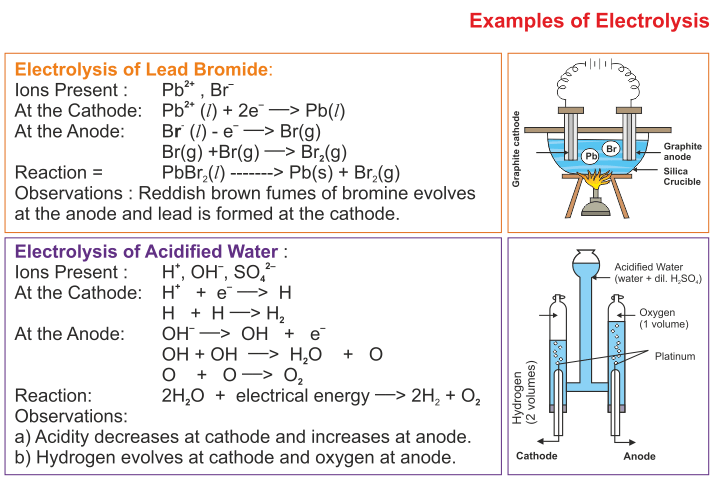Question 8: (i) Write equations to show the electrolytic dissociation of:
(a) Two acids (b) Two bases.
(ii) When fused sodium chloride is electrolyzed, explain exactly what happens at the electrodes and explain how the electricity is conducted?
H2SO4   ⇌   2H+ + SO42-
HNO  ⇌   H+ + NO3
(b) Bases:
NaOH    ⇌    Na+ + OH
KOH    ⇌    K+ + OH
(ii) When electricity is passed through fused sodium chloride, the electrolysis starts as follows:
NaCl    ⇌    Na+ + Cl
Na+ + e–   ⟶   Na (At cathode)
Cl – e  ⟶   Cl
Cl + Cl   ⟶  Cl2 (At anode)
Sodium metal is deposited at the cathode, while chlorine gas is liberated at the anode. Electricity is conducted with the help of free sodium and chloride ions, which are present infused sodium chloride.

Question 9: How is it possible to discharge Na+ ions in preference to H+ ions in the electrolysis of NaCl solution?
Answer: By using a cathode made of moving mercury, Na+ ions are discharged in preference to H+ ions because of the nature of the electrode. Mercury has a strong tendency to form an amalgam with sodium
Na+ + e–   ⟶   Na
Na + Hg   ⟶  Na/Hg
When the sodium amalgam dissolves in water, the reaction is
2Na/Hg + 2H20   ⟶  2NaOH + H2 + Hg

Question 10: A certain metal, say M, does not liberate hydrogen from dilute sulphuric acid, but displaces copper form aqueous copper (II) sulphate. State the most likely place for the metal in the electrochemical series.
Answer: The activity series is obtained when we examine the replacement of one metal ion from its solution by another metal. The metal (M) which displaces copper from aqueous copper (II) sulphate is placed at a higher position as compared to copper in the activity series.

Question 11: M is a metal above hydrogen in the activity series and its oxide has the formula M2O. This oxide when dissolved in water forms the corresponding hydroxide which is a good conductor of electricity. In the above context answer the following:
(i) What kind of combination exists between M and O ?
(ii) How many electrons are there in the outermost shell of M ?
(iii) Name the group to which M belongs.
(iv) State the reaction taking place at the cathode.
(v) Name the product at the anode.
Answer: (i) Electrovalent bond exists between M and O.
(ii) One electron is there in the outermost shell.
(iii) M belongs to First group.
(iv) M+ + e  ⟶   M (at cathode).
M + M   ⟶  M2
(v) Oxygen gas is liberated at anode.

Question 12: How will you electrolyze the molten solution of lead bromide?
Answer: Molten lead bromide (PbBr2) forms and Br ions. The positive lead ions (Pb2) move to the cathode, and gain two electrons and change into the lead atom.
Pb2+ + 2e–   ⟶   Pb (Lead)
Lead is deposited at the cathode. The negative bromide ion migrates to the anode. It loses an electron and becomes a bromine atom. The two bromine atoms join the bromine molecule. It is liberated as bromine gas.
Br – e   ⟶   Br
Br+Br   ⟶   Br2

Question 13: (i) What are the particles present in a non-electrolyte?
(ii) What is the conductivity of metals due to?
(iii) What should be the physical state of lead bromide, if it is to conduct electricity?
(iv) What particles are present in pure lead bromide?
Answer: (i) Molecules are present in a non-electrolyte.
(ii) The conductivity of metals is due to the movement of electrons.
(iii) Lead bromide should be in molten state if it is to conduct electricity.
(iv) Lead ions and bromide ions are present in pure lead bromide in molten state.

Question 14: (i) When the electrolysis of acidified water is carried out:
(a) What is the ratio of the volume of hydrogen produced to the volume of oxygen?
(b) Give the equation for the discharge of ions at the cathode.
(ii) To carry out the so-called “electrolysis of water”, sulphuric acid is added to water. How does the addition of sulphuric acid produce a conducting solution?
Answer: (i) (a) The ratio is 2 : 1
(b) H+ + e   ⟶   H; 2H + 2H   ⟶   2H2
(ii) Addition of sulphuric acid causes dissociation of water molecules into [H+] and [OH] ions.

Question 15: What would happen if in the electrolysis of acidified water, copper electrodes were used instead of platinum ones ?
Answer: At anode : OH and S032- would migrate to anode but neither would be discharged, instead copper atoms would get oxidised to Cu2+ and enter solution
Cu   ⟶   Cu2+ + 2e
The electrolytic solution would become blue in colour. Anode would dissolve.
At cathode : H+ ions would migrate to the cathode and get reduced. Thus, H2 gas would be discharged at cathode
2H+ + 2e   ⟶   2H   ⟶   H2
Later, as the solution turns blue due to formation of Cu++ ions, the Cu++ ions will get discharged to 2Hions as they are less electropositive
Cu2+ + 2e   ⟶   Cu↓

Question 16: During the electrolysis of aqueous copper sulphate, between copper electrodes, the sulphate and hydroxyl ions remain as spectator ions.
Answer: During electrolysis of aqueous copper sulphate using copper electrodes, the two anions OH.and S042- migrate to the anode, but none of them get discharged because the copper of the anode dissolves in the solution producing copper ions and electrons. Hence, OH and S042- ions remain as spectator ions.

Question 17: The following questions refer to the electrolysis of copper sulphate solution with copper electrodes.
(i) Compare the change in mass of the cathode with the change in mass of the anode.
(ii) What happens, when electrolysis of aqueous copper sulphate between platinum electrode, occurs.
(iii) What is the practical application of the electrolysis of copper sulphate solution? Briefly describe one such application.
Answer: (i) Mass of Cathode increases whereas that of anode decreases due to deposition of pure copper on cathode.
(ii) The blue colour of copper sulphate is due to the presence of cupric ions (Cu++). Cu++ ions are discharged at the cathode and deposited as Pinkish copper metal, but OH ions are discharged at anode. The electrolyte consists of hydrogen and sulphate ions which associate to form colourless sulphuric acid.
(iii) The electrolysis of copper sulphate solution is used in the purification of copper using pure copper plate as cathode and impure copper plate as anode.

Question 18: During the electrolysis of copper (II) sulphate solution using platinum as cathode and carbon as anode:
(i) What do you observe at the cathode and at the anode?
(ii) What change is noticed in the electrolyte?
(iii) Write the reactions at the cathode and at the anode.
Answer: (i) At cathode red shiny metal deposits.
At anode bubbles of a colourless odourless gas are seen coming out.
(iii) Reaction at cathode:
Cu2+ + 2e   ⟶   Cu
Reaction at anode:
OH – 1e  ⟶   OH
4OH   ⟶    2H2O + O2

Question 19: Explain, how the blue colour of electrolyte fades during electrolysis of CuSO4 solution?
Answer: The blue colour of electrolyte is due to the presence of copper ions in it. As the electrolysis is carried out, the copper ions discharge at the cathode.
Cu2+ + 2e   ⟶   Cu
However, no copper ions enters in the electrolyte from anode. Thus the concentration of copper ions goes on decreasing. This result in fading of blue colour. When copper ions completely finish the electrolyte becomes colourless.
Reaction of cathode:
H+ + e   ⟶   H
H + H   ⟶   H2
Reaction of anode :
OH – 1e  ⟶   OH
4OH   ⟶    2H2O + O2(g)

Question 20: Explain, how blue colour of electrolyte remains unchanged during electrolysis of aqueous copper sulphate ?
Answer: The decrease in the weight of copper anode is equal to the increase in weight of copper cathode.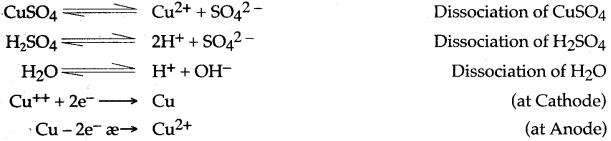From the above equations it can be deduced that for every Cu2+ discharging at cathode a copper atom ionises from anode to form Cu2+ ion.
Thus concentration of copper ions in electrolyte remain unchanged. As the blue colour of electrolyte is due to the presence of Cu++ ions, therefore it does not change.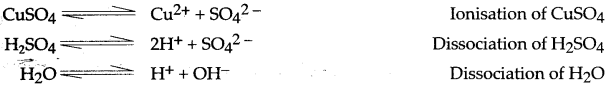Question 21: Mention the type of ions present, the products obtained and the electrode reactions that occur, when the following are electrolysed :
(i) Molten lead bromide between steel cathode and a graphite anode.
(ii) Water acidified with sulphuric acid between platinum electrodes.
(iii) Aqueous copper sulphate between copper electrodes.
(iv) Aqueous copper sulphate between copper cathode and platinum anode.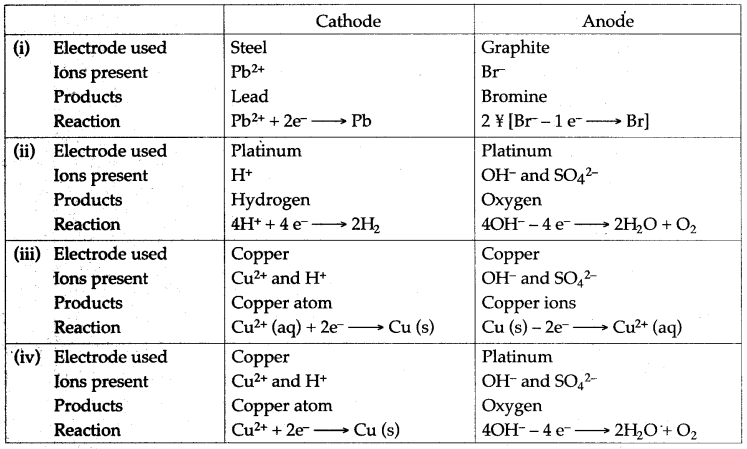Question 22: With reference to electroplating answer the following :
(i) Why are articles electroplated?
(ii) Why a small current passed for a longer period is preferred over high current for a shorter period?
(iii) Why the article to be electroplated is made a cathode?
(iv) Why a direct current is used ?
Answer: (i) Articles are electroplated for the following reasons:
(a) To prevent it from corrosion.
(b) To improve the appearance of the metal particles.
(ii) To get a uniform and smooth coating of superior metal, a small current should be used -for a longer time.
(iii) The article to be electroplated is always placed at cathode because the metal is always deposited at the cathode.
(iv) Direct current is used to get smooth coating and the phase of the current is same at all instances of time.

Question 23: How a spoon is electroplated with silver?
Answer: Silver nitrate solution is taken in a beaker. Silver wire and a spoon, which is to be plated with silver, are dipped in the solution. Silver wire is connected with the positive terminal of the battery which acts as anode and spoon is connected with the negative terminal of the battery which acts as a cathode.
When electric current is passed through silver nitrate solution, electrolysis takes place and silver is deposited as a fine thin film at the surface of spoon. The spoon is coated with silver and looks like a silver spoon.

Question 24: Element X is a metal with a valency 2.
Element Y is a non-metal with a valency 3.
(i) Write equations to show how X and Y form ions.
(ii) If Y is a diatomic gas, write the equation for the direct combination of X and Y to form a compound.
(iii) Write two applications of electrolysis in which the anode diminishes in mass.
(iv) If the compound formed between X and Y is melted and an electric current passed through the molten compound, the element X will be obtained at the … and Y at the … of the electrolytic cell.
(Provide the missing words.)
Answer: (i) X – 2e   ⟶   X2+
Y + 3e   ⟶   Y3-
(ii) 3X+2 + 2Y-3   ⟶   X3Y2
(iii) (1) Electroplating of Metals.
(2) Electro-refining of Metals.
(iv) If the compound formed between X and Y and an electric current passed through the molten compound, the element X will be obtained at the cathode and Y at the anode of the electrolytic cell.

Question 25: Mr. Ramu wants to electroplate his key chain with nickel to prevent rusting. For this electroplating :
(i) Name the electrolyte
(ii) Name the cathode
(iii) Name the anode
(iv) Give the reaction at the cathode
(v) Give the reaction at the anode.
Answer: (i) Nickel sulphate (ii) Key chain
(iii) Pure nickel plate
(iv) Ni2+ + 2e  ⟶   Ni
(v) Ni – 2e   ⟶   Ni2+.

Question 26: Three different electrolytic cells A, B, and C are connected in separate circuits. Electrolytic cell A contains sodium chloride solution. When the circuit has completed a bulb in the circuit glows brightly. Electrolytic cell B contains the acetic acid solution and in this case the bulb in the circuit glows dimly. The electrolytic cell C contains sugar solution and the bulb does not glow. Give a reason for each of these observations.
Answer: In Cell A: Sodium chloride being a strong electrolyte dissociates completely and therefore current flows better.
In Cell B: Acetic acid being weak electrolyte ionises only partially and therefore, only a weak current flows.
In Cell C: Sugar being a covalent compound does not ionise at all and therefore, no current flows.

### Figure/Table Based Questions

Question 1:(i) Name the cathode and anode used during electroplating silver.
(ii) Name the electrolyte used in this process.
(iii) Give the dissociation reactions taking place.
(iv) Give the reactions occurring at cathode and anode.
(y) The overall strength of silver ions remains constant in the reaction. Why?
Answer: (i) Cathode: Highly cleaned article such as copper cup.
Anode : A plate or rod of silver.
(ii) Sodium argento cyanide (Na[Ag(CN)2]) and hydrocyanic acid (HCN).(v) Overall strength of silver ions does not change in the electrolyte as the number of Ag+ ions entering the electrolyte is equal to the number of Ag+ ions discharged at cathode.

Question 2: Copper sulphate solution is electrolysed using copper electrodes. Study the diagram given below and answer the question that follows:(i) Which electrode to your left or right is known as the oxidising electrode and why?
(ii) Write the equation representing the reaction that occurs.
(iii) State two appropriate observations for the above electrolysis reaction.
Answer: (i) Electrode on the left side is the oxidising electrode because copper atoms lose electrons at this electrode.
(ii) Cu – 2e–  ⟶  Cu2+
(iii) Reddish-brown copper metal is deposited at cathode and blue colour of aqueous copper (II) Sulphate solution remains unchanged.

Question 3: (i) Study the diagram given below and answer the questions that follows :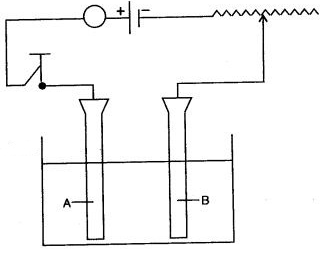(a) Give the names of the electrodes A and B.
(b) Which electrode is the oxidizing electrode?
(ii) A strip of copper is placed in four different colourless salt solutions. They are KNO3, AgNO3, Zn(NO3)2, Ca(NO3)2. Which one of the solutions will finally turn blue?
(iii) Write the equations of the reactions which take place at the cathode and anode when acidified water is electrolysed.
B—Cathode
(b) A
(ii) AgNO3 solutionQuestion 4: Study the given figure and answer the question that follow :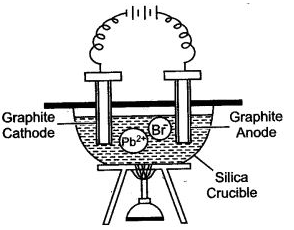(i) Why silica crucible is used in this type of electrolysis?
(ii) Which anode is preferred and why?
(iii) Why crucible is heated from the outside?
(iv) Write the equations of the reaction which take place at the cathode and anode.
Answer: (i) Silica is non-reactive. It can withstand high temperatures and is almost a non-conductor of electricity.
(ii) The graphite anode is preferred because it is unaffected by the reactive bromine vapors.
(iii) The crucible is heated from outside to keep lead bromide in the molten state. So that the ions become free.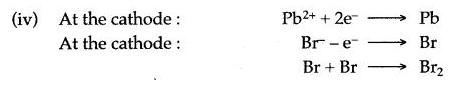Question 5: An electrolytic all is a set up using two platinum electrodes and an aqueous solution of copper (II) sulphate.
(i) Draw a labelled diagram of the electrolytic cell.
(ii) Name the ions present in the cell.
(iii) Name the ions migrating towards the anode.
(iv) Name the ios migrating towards the cathode.
(v) Name the ions which will not be discharged at electrodes during electrolysis.
(vi) Write the reaction at the cathode.
(vii) Write the reaction at the anode.
(viii) Name the spectator ions in the solution.
(ix) Why the electrolytic solution acidified.(ii) The ions present in the cell are Cu2+ + H+, SO42- and OH.
(iii) The ions migrating towards the anode are SO42- and OH.
(iv) The ions migrating towards the cathode is Cu2+.
(v) The ions will not be discharged at electrode during electrolysis are H+ and OH.(viii) The separator ions present in the solution are – SO42- and OH.
(ix) To increase the electrical conductivity of electrolyte.

Question 6: A saturated aqueous copper (II) chloride is electrolysed using graphite anode and copper cathode as illustrated in diagram given below: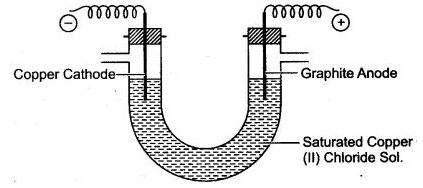(i) Name the ions which will migrate to cathode.
(ii) Nametheions which will migrate to anode.
(iii) Which ion is likely to discharge at cathode and why?
(iv) Write ionic equation fòr reaction taking place atcathode.
(v) Which ion is likely to discharge at anode and why ?
(vi) Write ionic equation for reaction taking place at anode.
(vii) If electric current is passed for a very long time, state colour change taking place in electrolyte. State one reason for the change.
(viii) Give one reason for using graphite anode, rather than copper anode.
(ix) Name the gas liberated at cathode after the colour changes in electrolyte.
Answer: (i) Copper ions (Cu2+) and hydrogen ions H+ migrate to cathode.
(ii) Chloride ions (Cl) and hydroxyl ions OH migrate to anode.
(iii) Copper ions (Cu2+) are likely to discharge at cathode, because their position is lower than hydrogen ions H+ in electrochemical series.
(iv) Cu2+ + 2e–  ⟶  Cu
(v) Hydroxyl ions (OH) are likely to discharge at anode, because their position is lower than chloride ion (Cl) in electrochemical series.
(vi) 4OH – 4e  ⟶   2H2O + O2 (g)
(vii) The electrolyte gets decolourised. It is because, the blue colour of electrolyte is due to the presence of Cu2+ ions. As Cu2+ ions discharge at cathode, therefore their concentration in electrolyte decreases. Thus, gradually blue colour fades away.
(viii) In such a situation the copper atoms on copper anode ionise and enter into electrolyte. Thus, size of copper anode gradually decreases. This is not possible in case of graphite anode.
(ix) Hydrogen gas is liberated at cathode.

### Reasoning Based Questions

Question 1: Why are adds, bases and salts classified as electrolytes?
Answer: Acids, bases and salts are classified as electrolytes because these compounds dissociate into ions, conduct electricity and undergo chemical decomposition at the same time.

Question 2: Metals like potassium, calcium, sodium, etc., can be extracted only by electrolysis.
Answer: Metals like K, Ca, Na, etc., can be extracted only by electrolysis because conventional reducing agents such as coke, carbon monoxide, hydrogen do not supply sufficient energy to break ionic bonds between the active metals and their chlorides or oxides.

Question 3: Dilute acids are strong electrolytes. Why?
Answer: Dilute acids produce a large number of hydronium ions, so they behave like strong electrolytes.

Question 4: Sea water is a strong electrolyte. Why?
Answer: Sea water is a strong electrolyte because sodium chloride dissolved in it dissociates completely into free mobile ions.
Na+Cl-  ⇌  Na+ + Cl

Question 5: Copper is a good conductor of electricity, but it is a non-electrolyte. Why?
Answer: During metallic conduction, the chemical properties of copper are intact as it does not undergo chemical decomposition. Since, the flow of electricity only produces heat and energy and no new products are formed copper metal is thus a good conductor of electricity but is a non-electrolyte.

Question 6: A solution of ionic compound is an electrolyte, while that of the covalent compound is non-electrolyte?
Answer: The solution of ionic compound has free ions, which can migrate to cathode and anode and discharge. Thus, solution of ionic compound is good conductor of electricity and hence is an electrolyte.
However, a solution of covalent compound consists of only molecules and does not have any free ions, which could migrate to cathode or anode. Hence, it is non-electrolyte.

Question 7: Explain, why hydrochloric acid is a conductor of electricity?
Answer: Hydrochloric acid dissociates into ions in an aqueous solution as follows:
HCl   ⇌  H+ + Cl
When a current is passed through in an aqueous solution of HCl, the ions move towards their respective electrodes. Thus, hydrochloric acid is a conductor of electricity.

Question 8: Does wax conduct electricity? Give reason to justify your answer.
Answer: No, wax does not conduct electricity because wax, being a covalent compound, does not have positively or negatively charged ions which could not be weakened by heating or in an aqueous solution. Therefore due to the absence of free ions, wax does not conduct electricity.

Question 9: Mercury is a liquid and allows the flow of electricity, though it is not an electrolyte.
Answer: An electrolyte is a substance which on dissolving in water breaks up into positively and negatively charged ions. But mercury is a metal, so on dissolving in water, it can’t break up into cations and anions, When electric current passes through mercury it doesn’t undergo any decomposition and no new substance is formed. Electric current passes through mercury due to the presence of free electrons in its penultimate shell and not due to the formation of ions.
Hence, mercury is a metallic conductor and not an electrolyte.

Question 10: A solution of cane sugar does not conduct electricity, but a solution of sodium chloride is a good conductor.
Answer: The sugar cane solution is a covalent compound. When it is dissolved in water, does not dissociate to give free ions which could migrate to cathode or anode. Hence, sugar solution is bad conductor of electricity. The sodium chloride solution mainly consists of free sodium and chloride ions which could migrate to positively charged electrodes. Hence, solution of sodium chloride is good conductor of electricity.

Question 11: Why does blue colour of CuSO4 solution slowly disappear when iron rod is dipped in it ?
Answer: When an iron rod is dipped in CuSO4 solution, iron displaces copper from CuSO4 to form FeSO4 which is light green in colour.Question 12: During electrolysis high voltage is not favoured. Why?
Answer: During electrolysis high voltage is not favoured because the electrolytic conduction increases with rise in temperature, i.e., a decrease in resistance. An increase in resistance can only be obtained by applying low voltage during electrolysis.
Thus, only electricity is suitable as a reducing agent which provides unlimited amount of energy to break ionic bonds easily.

Question 13: While electrolysing concentrated sulphuric acid, the bulb glows very dimly but when diluted, the bulb glows brightly. Why?
Answer: Concentrated (99%) sulphuric acid behaves like a weak electrolyte as it has very few hydronium ions in it. But when added to water, it becomes diluted and produces a large, number of hydronium ions. Now, it behaves like a stronger electrolyte and hence the bulb glows brightly.

Question 14: Why electrolysis of acidulated water is considered an example of electrolysis?
Answer: It is because the amount of sulphuric acid does not change when water is electrolysed. The sulphuric acid just helps in increasing the conductivity of water.

Question 15: Why should water be acidified before proceeding with the electrolysis of water?
Answer: It is done because water is a non-electrolyte, so it can be electrolytically decomposed by removing the H+ and OH ions continuously because the negligible ionization of water yields H+ and OH ions which recombine to form a water molecule, then another molecule will ionize. Sulphuric acid is used to remove these ions.Question 16: Hydroxyl (OH) ion is lower in the activity series, than chloride ion. Yet when a concentrated solution of hydrochloric acid is subjected to electrolysis, the hydroxyl ion does not get discharged.
Answer: If an electrolyte has a much higher concentration of ions that are higher in the electrochemical series than those that are lower, then the higher gets discharged in preference to the lower one. Concentrated hydrochloric acid being strong electrolyte gets fully dissociated furnishing H+ and Cl ion. Water is less dissociated into H+ and OH ion. Thus, concentration of chloride ion is more as compared to OH ion and hence, chloride ion are discharged in preference to the OH ion.

### Balancing/Writing the Chemical Equations

Question 1: Write equations for the reactions taking place at the cathode and at the anode during the electrolysis of :
1. Acidified nickel sulphate solution with nickel electrode.
2. Acidified copper sulphate solution with the copper electrode.
3. Acidified copper sulphate solution with the platinum electrode.
4. Acidulated water with inert electrode.
5. Molten lead bromide with inert electrodes.
6. Electroplating a spoon with silver.